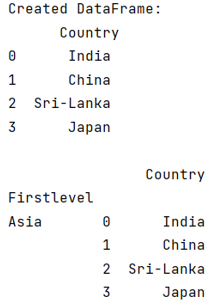# How to prepend a level to a pandas MultiIndex?

Given a Pandas MultiIndex, we have to prepend a level in it.
Submitted by Pranit Sharma, on June 19, 2022

Columns are the different fields that contain their particular values when we create a DataFrame. We can perform certain operations on both rows & column values.

Multilevel indexing is a type of indexing that include different levels of indexes or simply multiple indexes. The DataFrame is classified under multiple indexes and the topmost index layer is presented as level 0 of the multilevel index followed by level 1, level 2, and so on.

To learn how to prepend a level to pandas multiindex, we will use pandas.concat() method.

The pandas.concat() method is used to add any column or value in DataFrame based on the specified object passed inside the method. Here, we will pass our whole DataFrame as a value along with a key which we want to make a level for multi-index purpose.

To work with pandas, we need to import pandas package first, below is the syntax:

```import pandas as pd
```

Let us understand with the help of an example,

## Python code to prepend a level to a pandas MultiIndex

```# Importing pandas package
import pandas as pd

# Creating a dictionary
d= {'Country':['India','China','Sri-Lanka','Japan']}

# Creating a DataFrame
df = pd.DataFrame(d)

# Display Original DataFrame
print("Created DataFrame:\n",df,"\n")

# Prepend a level named as firstlevel
print(pd.concat({'Asia': df}, names=['Firstlevel']))
```

Output:Preparation

What's New

Top Interview Coding Problems/Challenges!